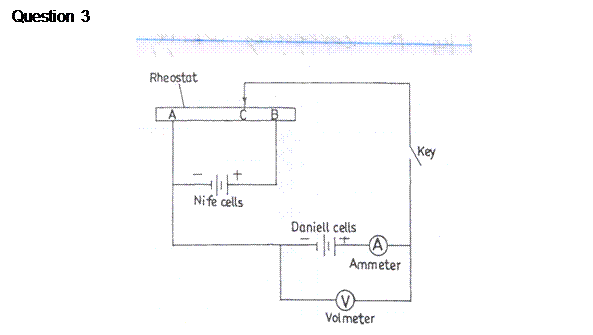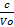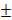Home Technical Mathematics Languages Science Social Science Art Literature Arabic Islamic Studies C.R.K History MusicVisual Art Clothing/Textile Home Management Shorthand
 Physics Paper 2, Nov/Dec. 2013
 Questions: 1 2 3 Main
Weakness/Remedies
Strength

QuestioN 2An electric circuit was set-up as shown in the diagram above.
The position of the sliding contact, C, was adjusted on the rhcostat, AB, such that the ammeter readingo was zero.  The corresponding voltmeter reading Vo was read and recorded.

The procedure was repeated for six other values of ammeter reading Ii
In each case, the corresponding value of the voltmeter reading, Vi was read and recorded.
Fig 3(a) and Fig.3(b) represent the ammeter and voltmeter readings respectively.

1. Read and record the values, Ii, where i = 1,2,3,4,5 and 6
2. Read and record the corresponding voltmeter readings, Vi, where i = 1,2,3,4,5 and 6
4. Plot a graph with V on the vertical axis and I on the horizontal axis
5. Determine the slope, s, of the graph and the intercept, c, on the vertical axis
6. Evaluate K =7. State two precautions that are necessary to ensure accurate results when performing this experiment.

(b)       (i)         State four factors which affect the resistance of a metallic conductor
(ii)        Define electromotive force.
This question was the most popular among the candidates and it was well attempted by most responding candidates.  The observations was well handled.  Graph of V against I was satisfactorily plotted.  Slope, intercept and deductions were well attempted however, few could not obtain the intercept from the graph because they did not start the graph from the origin (00,).
Evaluation of K =was well attempted except for few candidates that did not record Vo.
Precautions were well stated.  In part (b), the factors affecting the electrical resistance of a metallic conductor were well stated but majority of the candidates could not define correctly the term electromotive force.

_____________________________________________________________________________________________________

Candidates are expected to:
(i)    Read and record Vo  to at least 2 d.p and within tolerance of ± 0.005 V
(ii)   Read and record six  values of V correctly to 2 d. p and within
tolerance of0.005V
(iii)  Read and record  six values of I correctly to 1 d.p and within
tolerance of0.05 A

2. Plot a graph with V on the vertical axis and I on the horizontal axis using reasonable scales
3. Distinguish between the axes and draw line of best fit
4. Determine the slope, of the graph intercept and evaluate       K =5. State any two of the following precautions
1. Avoided error  due to parallax in reading ammeter / voltmeter
2. Removed key when reading is not being taken
3. Ensured tight connections/ clean terminals
4. Noted / corrected zero error on voltmeter / ammeter
6. State length; cross-sectional area/thickness; nature of material/resistivity and temperature as factor, affecting the resistance of a metallic conductor.
7. State electrometric force as follows:

Is the work done in taking a unit (positive) charge round a (closed) circuit in which the cell is connected.                                OR
Is the potential difference across the terminals of the cell when the cell is in open circuit.